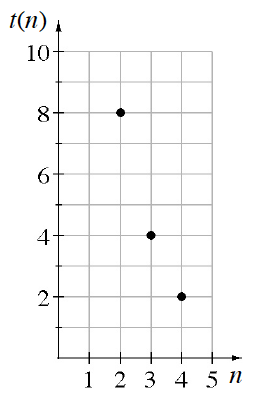### Home > CCAA8 > Chapter 6 Unit 7 > Lesson CCA: 6.2.1 > Problem6-56

6-56.

Write an explicit equation for the sequence graphed at right.

The multiplier for this graph is $\frac{1}{2}$

Work backward to find term $0$, or the starting value.

Use the starting value and the multiplier to write the equation.

$t(n)=32\left( \frac{1}{2} \right)^n$